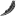What Is A Profit Margin & How To Calculate A Profit Margin

# What Is A Profit Margin & How To Calculate A Profit Margin

byThe profit margin is something that every business has, but not every business owner understands. Small business owners especially struggle with this concept. In this tutorial we will explain exactly what a profit margin is, how to calculate it, and how to use it in your business.

## Profit Margin Definition

The profit margin, also referred to as the net profit margin is a ration of ratio of profitability calculated as net income divided by revenues. It measures how much out of every dollar of sales a company actually keeps in earnings. In other words, the ratio you get from this calculation tell you how profitable your business operations are given how you currently conduct business.

A common way to evaluate a particular business is to look at the profit margin of a number of companies in the same industry and compare their profit margins. It is a sign of how well a company is run compared to similar companies.

Profit margin is typically displayed as a percentage. A 50% profit margin, for example, means the company has a net income of \$0.50 for each dollar of sales.

## How To Calculate The Profit Margin Ratio

As mentioned earlier, all you do to calculate the profit margin is:

Profit margin = total net profit / total revenue

## Operating Profit Margin

Another confusing term is the operating profit margin which is the measurement of how the proportion of revenue left after paying for variable costs of production such as wages, rent, fees, etc.

A healthy operating margin is required for a company to be able to pay for its fixed costs, such as interest on debt.

## Negative vs. Positive Profit Margin

by